# Black-Scholes Greeks Calculator

## Calculating Option Greeks with the Black-Scholes Calculator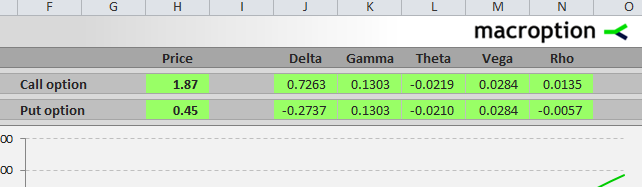When you calculate an option's price in the Black-Scholes Calculator, the Greeks will also be calculated at the same time, because they need the very same input parameters as option prices:

• Underlying price
• Strike price
• Volatility
• Interest rate
• Dividend yield
• Time to expiration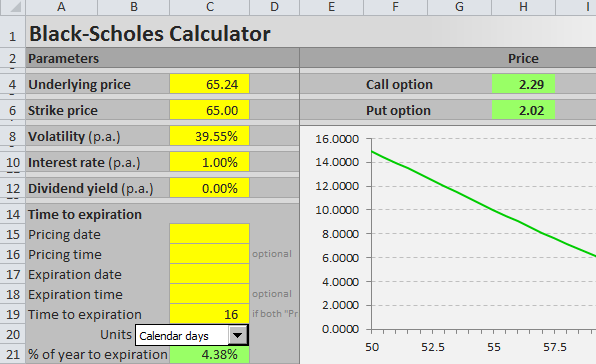You enter all the parameters in the top left corner of the "Pricing" sheet, in the area ranging cells A4-D21.

You can see the resulting option prices in column H and the Greeks – delta, gamma, theta, vega, and rho – in columns J-N, in row 4 for a call option and in row 6 for a put option.

## Black-Scholes Greeks Charts

You can also display charts showing the impact of any of the 6 input parameters on any of the Greeks.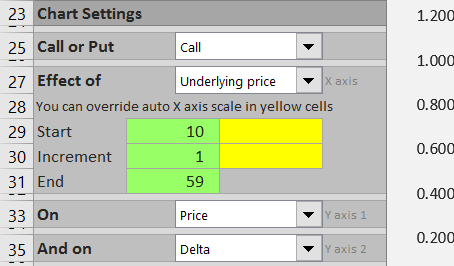Select call/put in the dropdown box in cell C25, the parameter in the combo in cell C27, and option price or any of the Greeks in combos in C33 and C35.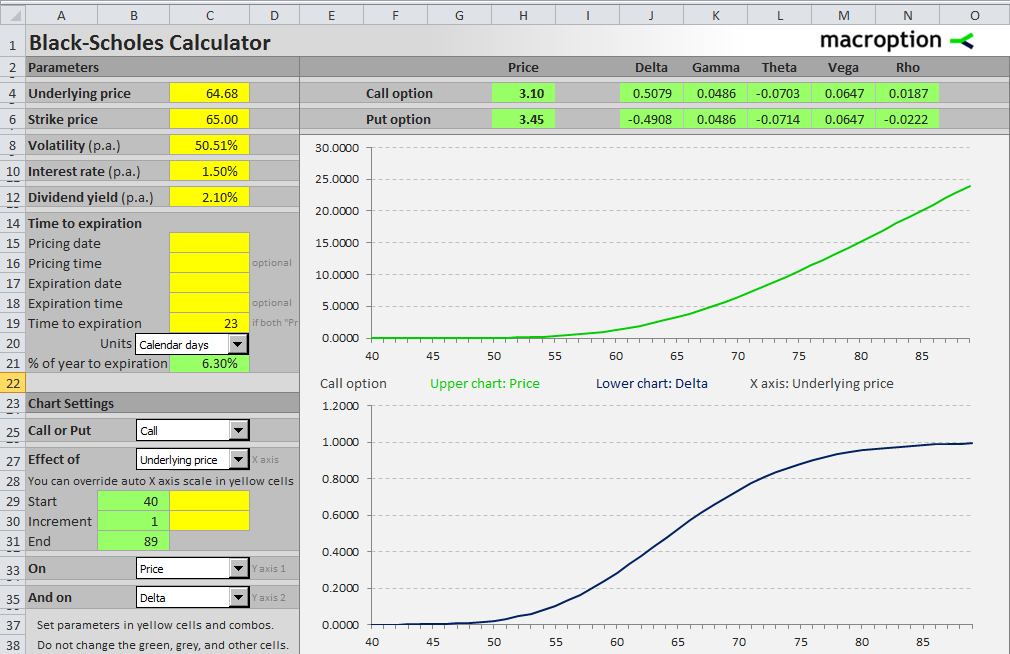On the screenshot above the charts show the impact of underlying price on a call option's price (upper chart) and delta (lower chart).If you want to see a smaller section of the price range in detail, you can adjust the X axis scale in cells C29 and C30.

## Black-Scholes Greeks Formulas

If you like, you can also see the exact Excel functions and calculations of the Greeks in the bottom part of the sheet "Pricing" (row 44 and below).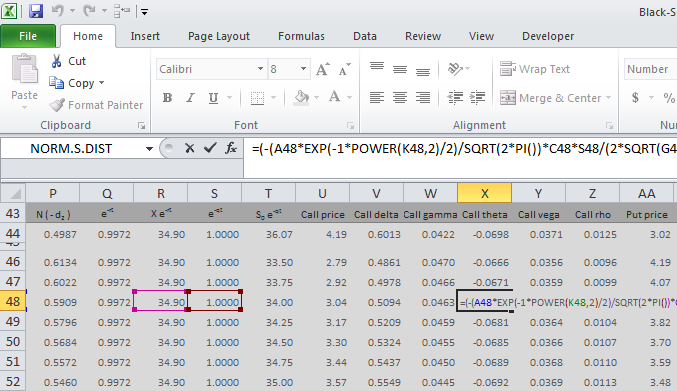The formulas and Excel calculations of call and put delta, gamma, theta, vega, and rho are explained in detail in chapters 9 and 10 of the user guide to the calculator.